# RS Aggarwal Solutions for Class 9 Chapter 1: Number Systems Exercise 1C

## RS Aggarwal Solutions for Class 9 Maths Exercise 1C PDF

By keeping in mind the major difficulties faced by the students to understand the concepts during the exam our subject experts provide solutions for each exercise in a step by step manner. Exercise 1C in Chapter 1 Number Systems mainly explains the rational numbers and the properties of rational numbers. The PDF of the solutions are made easy to download for the students which can be used to refer while solving the problems on a daily basis. RS Aggarwal Solutions for Class 9 Maths Chapter 1 Number Systems Exercise 1C are provided here.

## RS Aggarwal Solutions for Class 9 Chapter 1: Number Systems Exercise 1C Download PDF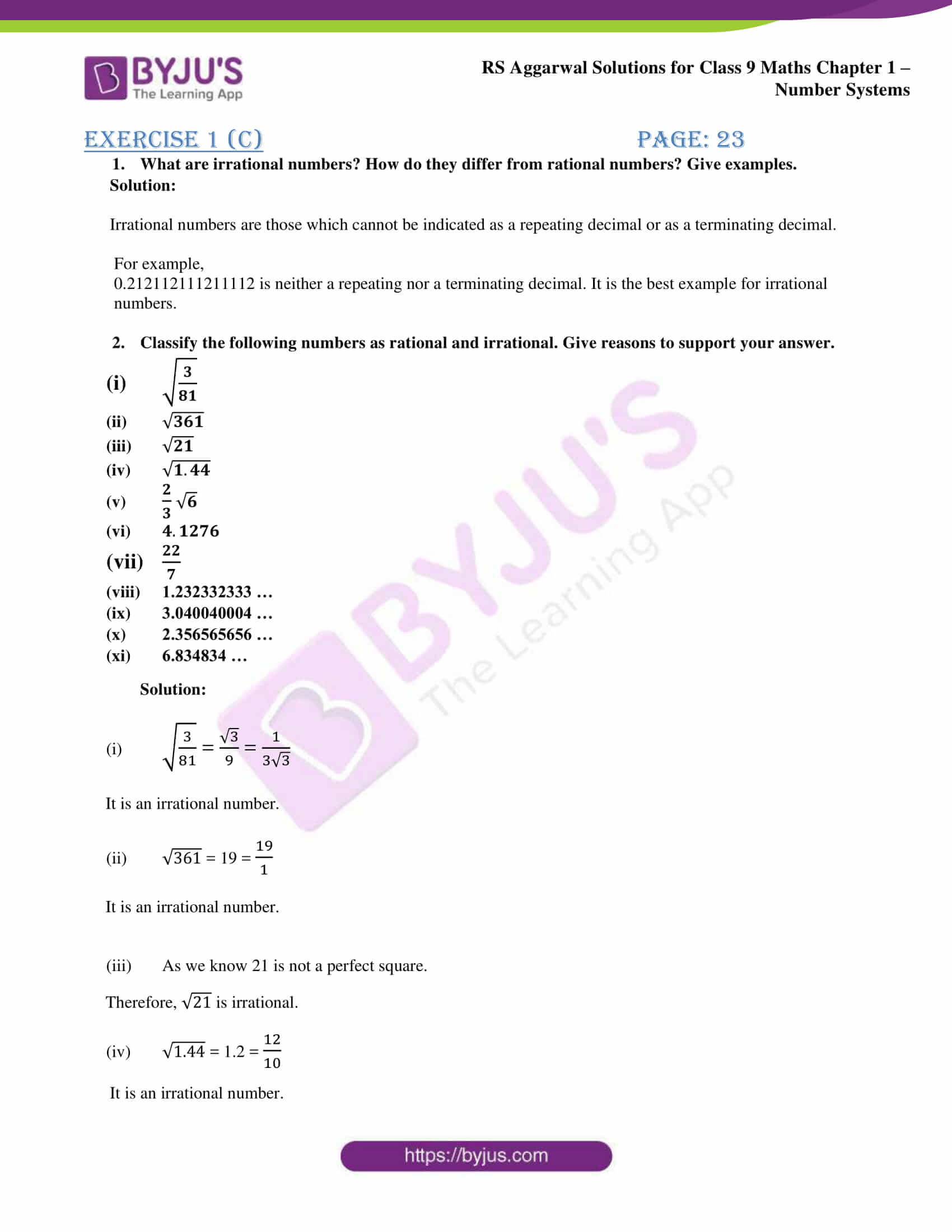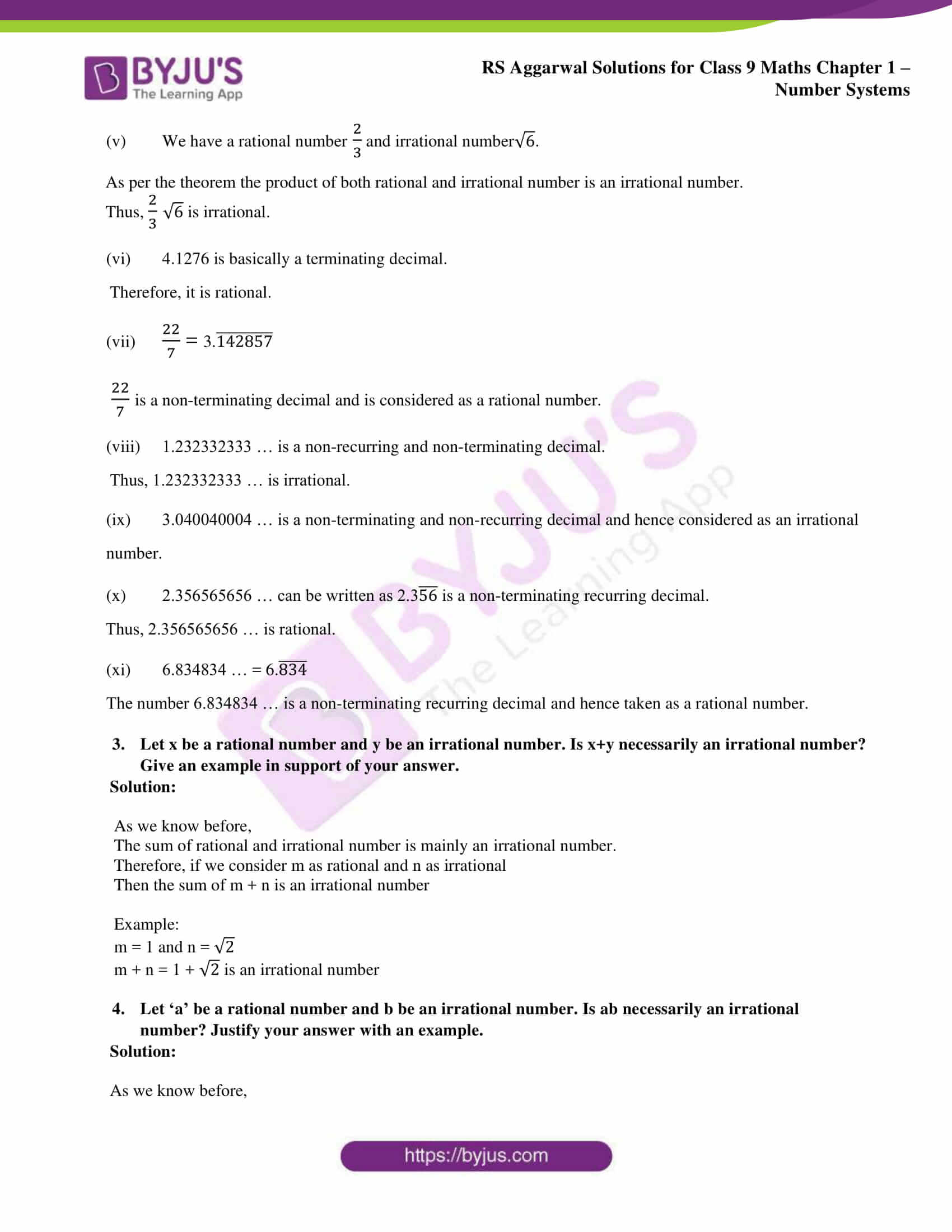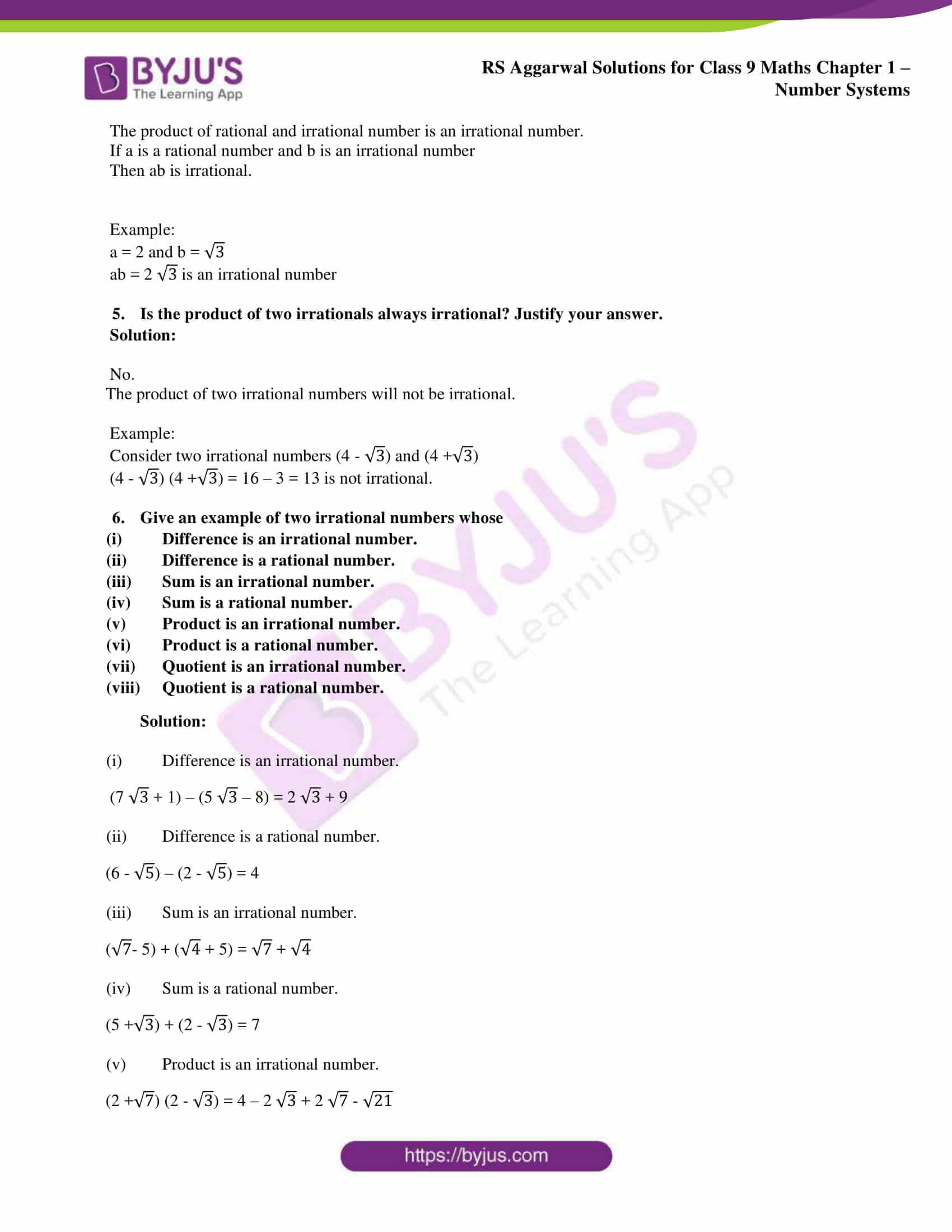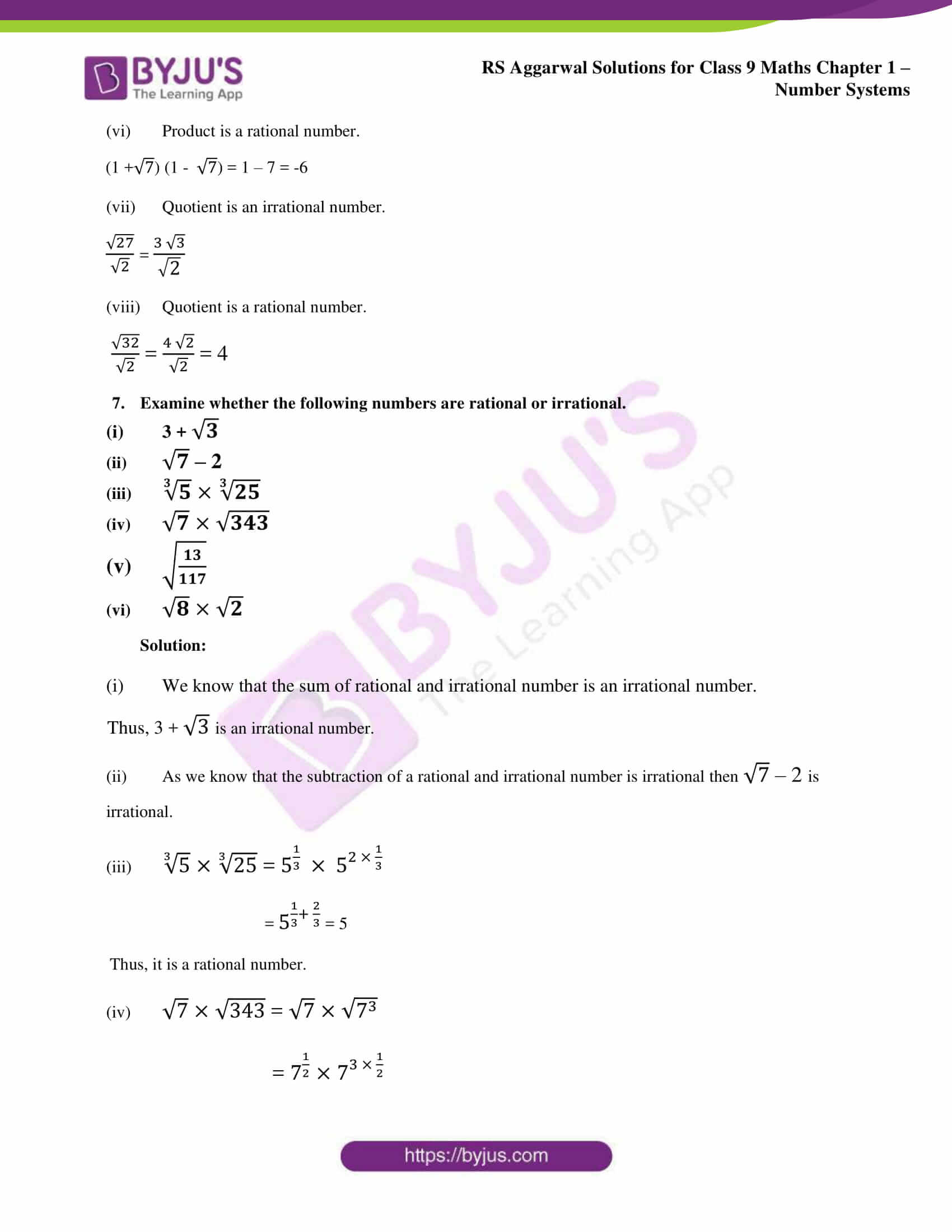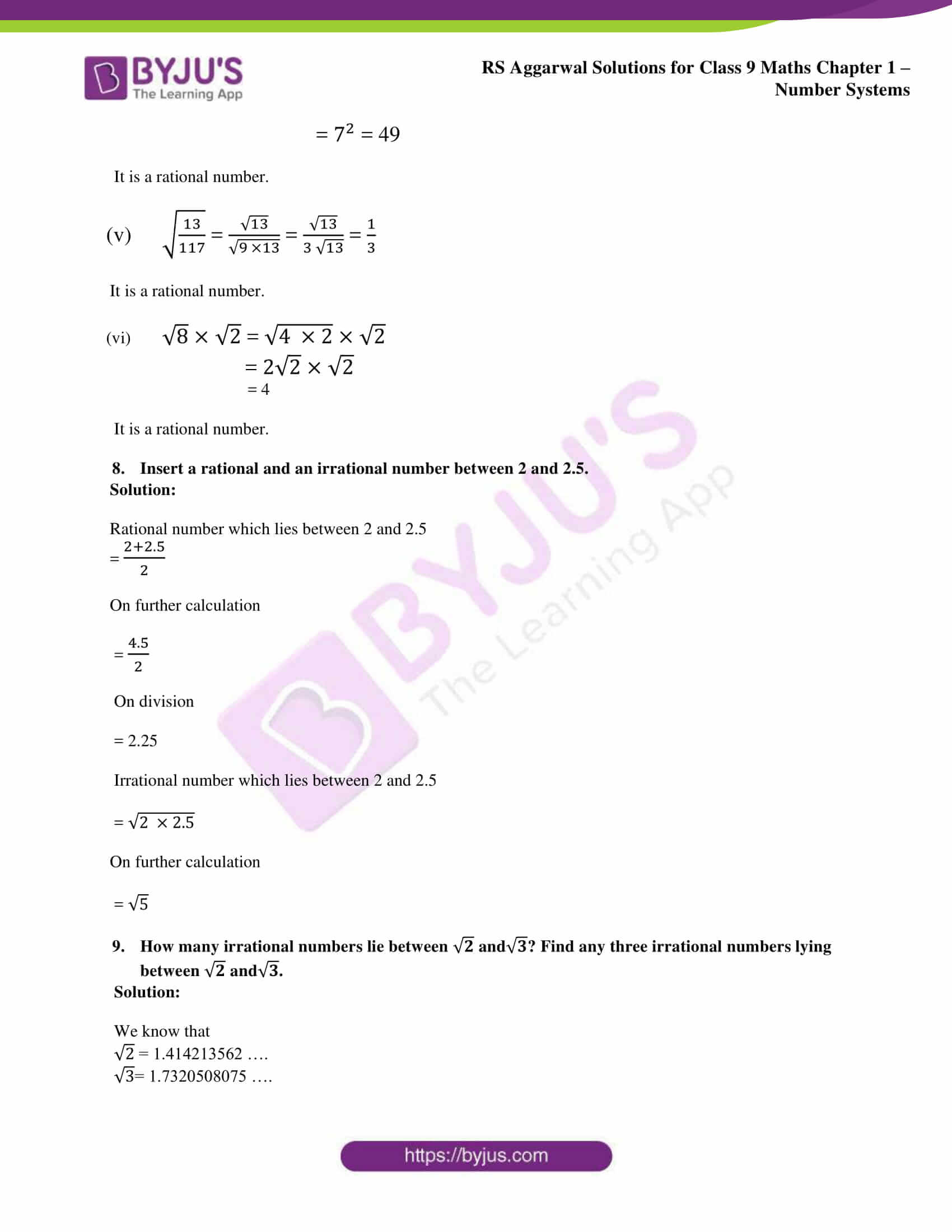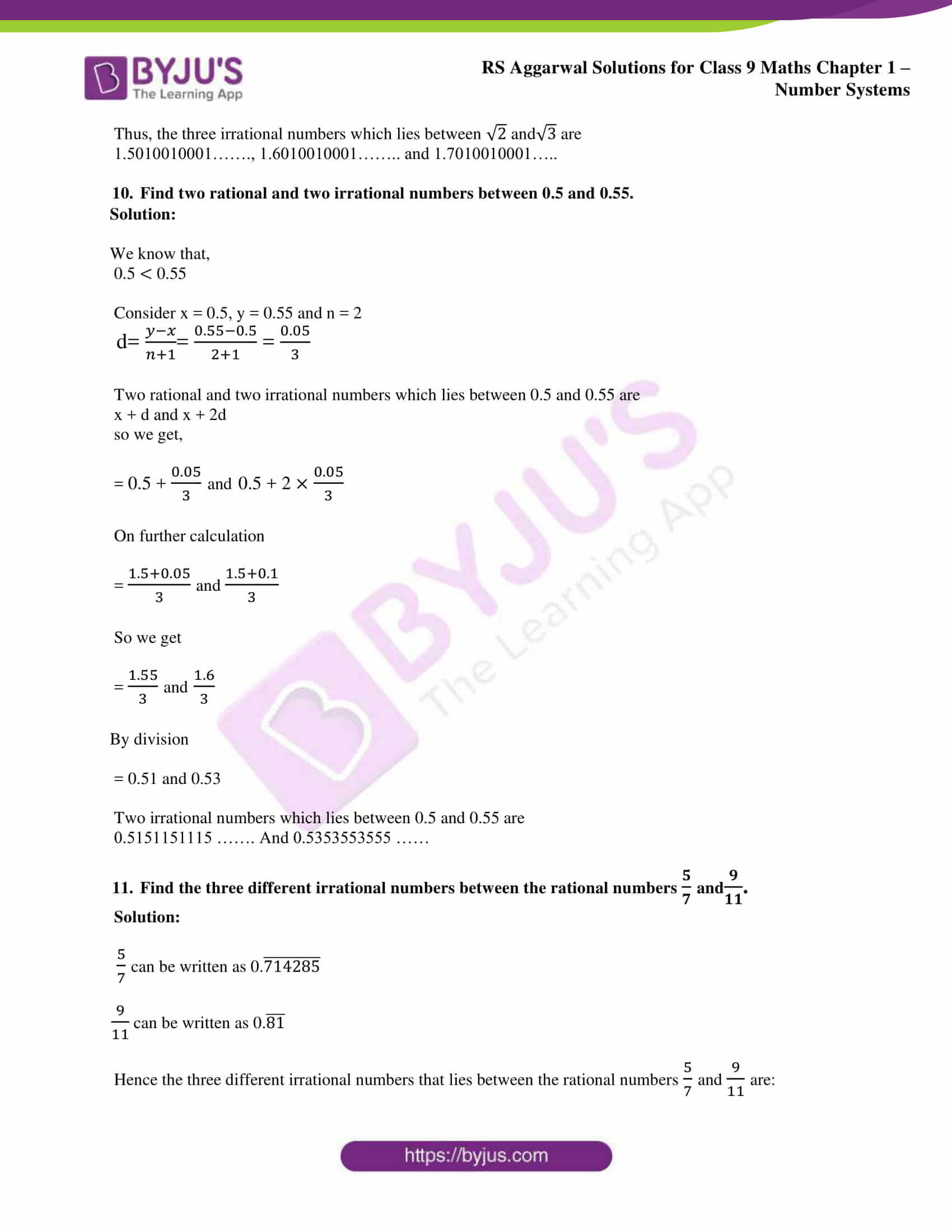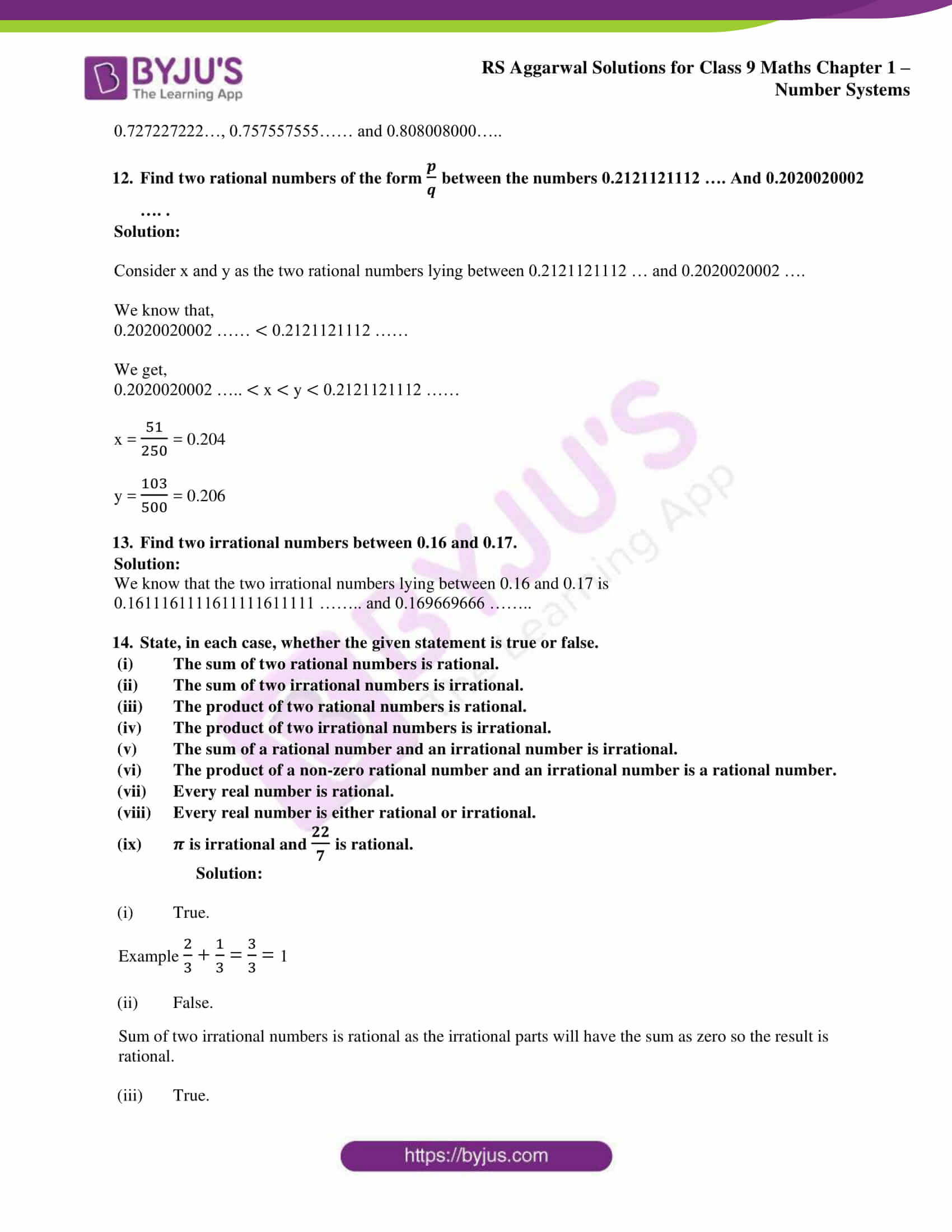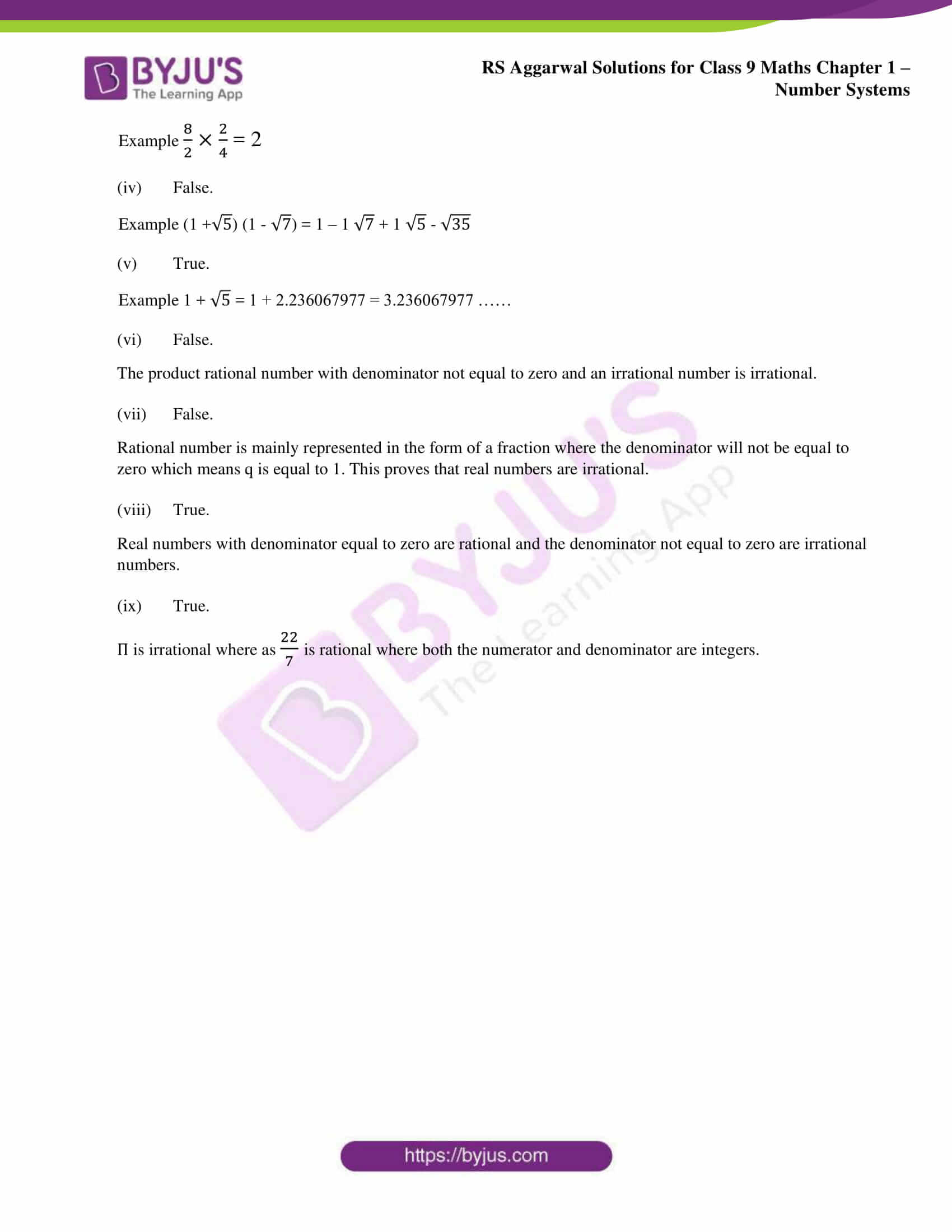### Access other exercise solutions of class 9 Maths Chapter 1: Number Systems

Exercise 1A Solutions 9 Questions

Exercise 1B Solutions 5 Questions

Exercise 1D Solutions 8 Questions

Exercise 1E Solutions 10 Questions

Exercise 1F Solutions 25 Questions

Exercise 1G Solutions 18 Questions

### RS Aggarwal Solutions Class 9 Maths Chapter 1 – Number Systems Exercise 1C

RS Aggarwal Solutions Class 9 Maths Chapter 1 Number Systems Exercise 1C has answers in an understandable language according to the students’ ability for topics like Rational numbers and their properties.

### Key features of RS Aggarwal Solutions for Class 9 Maths Chapter 1: Number Systems Exercise 1C

• RS Aggarwal Solutions for Class 9 mainly helps students to understand the major topics which are covered in each chapter.
• The solutions that are prepared by our expert faculties are based on the recent CBSE syllabus.
• It can be used as a reference guide by the students to clear the doubts which arise while solving the problems.
• The students can use the PDF in order to solve many number of problems according to the RS Aggarwal textbook and improve their problem solving abilities.